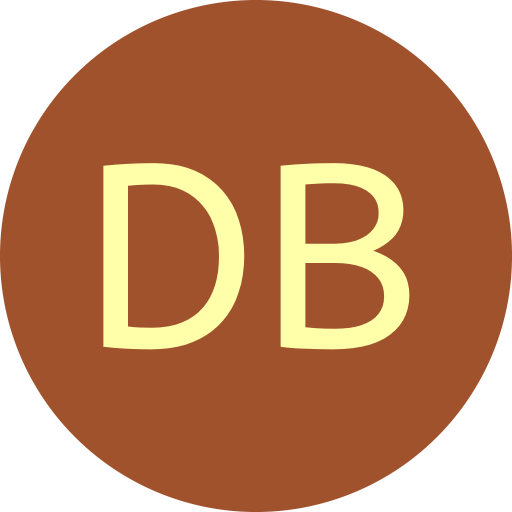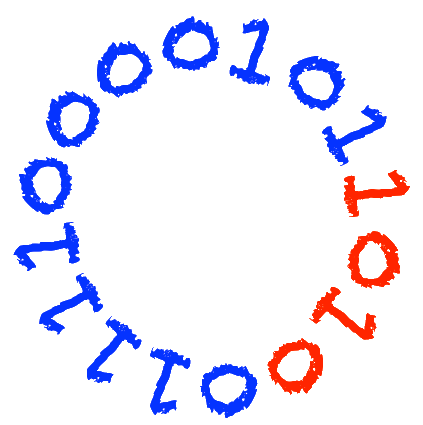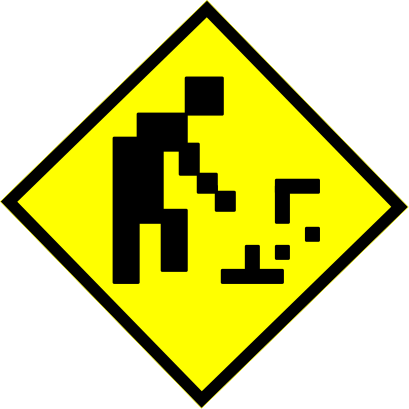#De Bruijn Sequence and Universal Cycle Constructions

A de Bruijn (DB) sequence is a circular string of length $k^n$ where every string of length $n$ over an alphabet of size $k$ appears exactly once as a substring.

 Example $n=4$ and $k=2$ 0000101101001111 is a DB sequence where the 16 unique substrings of length 4 visited in order are:   0000 0001 0010 0101 1011 0110 1101 1010   0100 1001 0011 0111 1111 1110 1100 1000The following different construction methods are presented:
• Greedy methods
• Shift rules (binary)
• Shift rules ($k$-ary)
• Concatenation approaches
• Euler cycle approach• LFSR and NFSRAdditionally, there is an algorithm to decode the lexicographically smallest DB sequence (the Granddaddy), an implementation of the cutting down variant, and information on the de Bruijn torus.

More generally, a universal cycle for a set $\mathbf{S}$ of length $n$ strings, is a circular string of length $|\mathbf{S}|$ where every string in $\mathbf{S}$ appears exactly once as a substring. Universal cycle constructions are provided for:

• Permutations (shorthand)
• Weak orders
• Weight range $k$-ary strings and relativesIt will be available soon via the public GIT repository